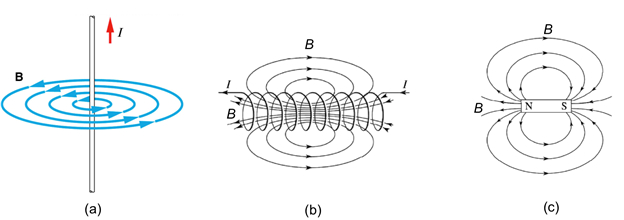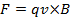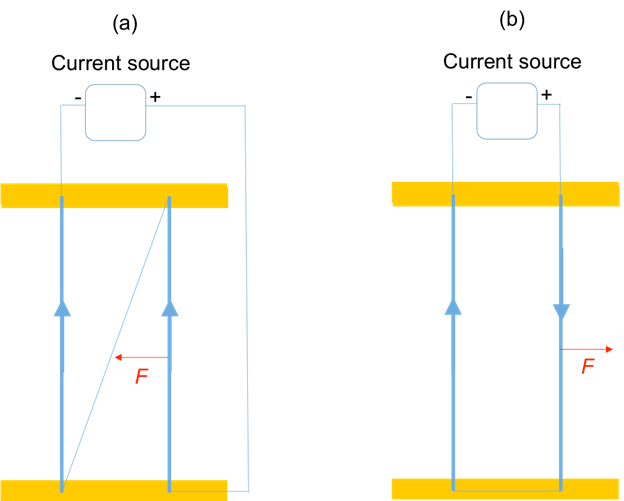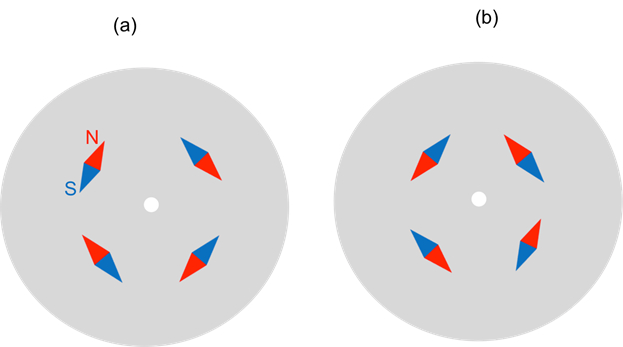Trial ends in

A subscription to JoVE is required to view this content.
You will only be able to see the first 20 seconds.

Magnetic Fields
Electric Fields
Capacitance

Magnetic Fields

Overview

Source: Yong P. Chen, PhD, Department of Physics & Astronomy, College of Science, Purdue University, West Lafayette, IN

Magnetic fields can be generated by moving charges, such as an electrical current. The magnetic field generated by a current can be calculated from the Maxwell equation. In addition, magnetic objects such as bar magnets can also generate magnetic fields due to microscopic dynamics of charges inside the material. Magnetic fields will exert magnetic force on other moving charges or magnetic objects, with the force proportional to the magnetic field. Magnetic fields are fundamental to electromagnetism and underlie many practical applications ranging from compasses to magnetic resonance imaging.

This experiment will demonstrate magnetic fields produced by a permanent bar magnet as well as an electrical current, using small compass needle magnets that align with magnetic fields. This experiment will also demonstrate the force exerted by the magnetic fields produced by a current on another current-carrying wire.

Principles

Magnetic fields (commonly referred to as "B fields") can be produced by moving charges (such as an electrical current) or "permanent magnets" (such as common bar magnets) made of magnetic materials (such as iron). If one follows the local direction of the magnetic field to traces out the vector field lines, these lines (whose tangent reflects the local direction of magnetic field, and the density of the lines reflects the strength of the local magnetic field) are known as "magnetic field lines". They are fictitious lines that help visualize the distribution and direction of magnetic fields.

For example, a long straight wire carrying an electrical current I will produce a magnetic field in the surrounding space: the magnitude of the magnetic field is proportional to the current I and inversely proportional to the distance r from the wire; and the direction of the magnetic field (represented by "magnetic field lines") is along the circular tangent direction around the wire (determined by the so-called "right-hand rule", with the thumb pointing along the current and the fingers curling around the magnetic field direction), depicted in Figure 1a. A solenoid (made of many turns of current loops or coils) will produce a magnetic field that is also proportional to the current in the coil, and mostly uniform and along the long axis inside the solenoid (also determined by the right-hand rule, with fingers curling around the current and thumb pointing along the magnetic field), but spreads out and decays outside the solenoid (the magnetic field lines will return to the other end of the solenoid), depicted in Figure 1b. The magnetic field pattern produced by a bar magnet is similar to that by a solenoid, with the magnetic field lines leaving the north pole of the magnet and entering the south pole of the magnet, as depicted in Figure 1c.Figure 1: Diagram showing magnetic field patterns (visualized by magnetic field lines) generated by a straight-line current (a), a solenoid (b), and a bar magnet (c).

A magnetic field (B) will act on other magnetic objects and moving charges. A small bar magnet (such as a compass needle) placed in a magnetic field would tend to be aligned with the local magnetic field (meaning the south-north axis of the bar magnet is along the direction of the local magnetic field, which is also how the compass needle works to detect the direction of Earth's magnetic field). A magnetic field will exert a Lorentz force on a moving charge. The force is proportional to the local magnetic field (B), the charge (q), and its velocity (v), and points in a direction perpendicular to both the motion and the magnetic field. The Lorentz force vector (F) is proportional to the vector product between v and B and is given by:Therefore, when F is zero, the direction of motion is parallel to the magnetic field but otherwise would bend the trajectory of the motion of the charge. Because of the Lorentz force, a magnetic field also exerts a force on a current carrying wire (as long as the current is not parallel to the direction of the magnetic field).

Procedure

1. Visualize Magnetic Field Lines

1. Obtain a segment of straight conducting wire (at least several cm long), and a DC current source.
2. Obtain a plate with a hole in the middle and several small compass needles on the plate, each mounted on a pin and freely rotatable.
3. Feed the conducting wire through the hole so the straight section is perpendicular to the plate. Connect the conducting wire to the DC current source, as shown in Figure 2a. The connection can be made by cables with clamps.
4. Turn on the current source and supply +5 A of current in the wire. Observe the behavior of the compass needles.
5. Reverse the current to −5 A and observe the behavior of the compass needle again.
6. Disconnect and remove the conducting wire and the current source. Obtain and bring a permanent bar magnet with its long-axis parallel to the board, to approach the plate from the side, with the north end of the magnet closer to the plate, as shown in Figure 2b. Observe the behavior of the compass needles.
7. Flip the orientation of the bar magnet, with now its south end closer to the plate. Observe the behavior of the compass needles.Figure 2: Diagram showing experimental setups using (a) a current flowing in a straight segment of wire that runs perpendicular to a plate and through the center hole on the plate; or (b) a bar magnet brought close to the plate and oriented perpendicular to the plate, to generate magnetic fields, which will orient compass needles on the plate along the direction of the local magnetic fields.

2. Effect of Magnetic Field

1. Obtain two long and parallel conducting wires anchored on a framework, as shown in Figure 3a. If needed, one can use a wood frame with two parallel bars (top and bottom) and anchor or tape the two ends of the wires to the two bars.
2. Use cables and clamps to connect the two wires in series and to the power source, with the top end of one wire connected to the bottom end of the other wire, as shown in Figure 3a.
3. Turn on the power source so that current will flow in the same direction (flowing from top to bottom) in the two wires. Observe the two wires when the current is on.
4. Turn off and disconnect the power source, now reconnect the two wires such that they are now connected again in series to the source, but with the top ends of the two wires shorted, as shown in Figure 3b.
5. Now turn on the power source and observe that the current will flow through the two wires in opposite directions. Observe the two wires.Figure 3: Diagram showing experimental setups of two parallel wires with current flowing in the same (a) or opposite (b) directions.

Magnetic fields are fundamental to electromagnetism and underlie many practical applications ranging from compasses to magnetic resonance imaging.

Magnetic fields or B-fields can be generated by moving charges, such as an electrical current, or objects such as bar magnets due to the microscopic dynamics of charges inside the magnetic material.

This video will illustrate how to visualize magnetic fields produced by a current-carrying conductor and a permanent bar magnet. Moreover, this video will also demonstrate the force exerted by the magnetic fields produced by a current on another current-carrying wire.

Magnetic fields can be visualized using magnetic field lines. These are fictitious lines that help understand the distribution and direction of magnetic fields.

The tangent from a magnetic field line reflects the local direction of the magnetic field, and the density of the lines mirrors the strength of the local magnetic field, which in case of a bar magnet decreases as we move away from its surface. Different current conductor configurations produce different variations in magnetic field distributions.

For example, a long straight wire carrying an electrical current produces a magnetic field, whose direction, as represented by "magnetic field lines", is along the circular tangent direction around the wire.

In the case of a bar magnet, the magnetic field lines leave the north pole of the magnet and enter the south pole of the magnet. This is similar to the magnetic field pattern produced by a solenoid, which is a cylindrical coil of wire that is carrying current.

The direction of the magnetic field produced by a current can be determined by the "right-hand rule". The rule states that if the thumb points along the direction of the current, the fingers curling around the conductor indicate the direction of the magnetic field. Thus, a bar magnet, when brought close to the conductor, aligns with the generated local magnetic field.

Now we know that magnetic fields, produced by any conductor or magnet, interact with nearby magnetic materials. Additionally, the generated magnetic fields also interact with moving electrical charges, like those found in a second current carrying conductor.

When a moving charge 'q' is introduced in a magnetic field 'B', the field exerts a force 'F' on the charge. This is called the Lorentz force. The force is proportional to the magnetic field 'B', the charge 'q' and its velocity 'v', and is determined by the vector product of the velocity of the charge and the magnetic field, times the charge. The force therefore points in a direction perpendicular to both the motion of the charge and the magnetic field determined by the "right hand thumb rule".

Having reviewed the basics of magnetic fields, let us perform a simple experiment to visualize these magnetic field lines and demonstrate how the Lorentz force exerted by a generated magnetic field affects a parallel current-carrying wire.

Gather the necessary materials and instruments, namely a DC current source, a plastic board mounted with several compass needles and a straight conducting wire passing through its center, and a permanent bar magnet.

Observe the plastic board with a hole in its center. It is mounted with several compass needles around the center hole using pins, such that the needles are free to rotate.

Also note that the conducting wire is fed through the center hole of the board. Make sure that the wire is perpendicular to the board. Connect the wire to the DC current supply using cables with clamps.

Turn ON the current source and set the current supply to +5 amperes. Observe the behavior of the compass needles.

Next, turn OFF the power supply and switch the positive and negative cables. Then, turn ON the power supply to reverse the direction of the current flowing through the wire and observe the compass needles again.

Now turn OFF and disconnect the current supply and obtain a similar plastic board mounted with magnetic needles, but without the conducting wire fed through it. Next, identify the north pole of the bar magnet.

With the long-axis of the bar magnet parallel to the board, bring the north pole closer to the board from the side. Observe the compass needles for any change in orientation.

Now flip the bar magnet such that the south pole is closer to the board. Once again, observe the compass needles for any change in orientation.

First assemble a frame with two bars, one of them is horizontal running along the top of the frame and the other is vertical that connects the base to the first bar. Next, anchor or tape the mid section of the two long conducting wires to the frame. Dangle one end of both wires from the frame such that the two wires are parallel to each other.

Now, connect the end of the two wires to the switch and the terminals. Then connect the setup to a battery.

Make sure that the wires are connected such that the current flows in the same direction in both wires. Then, flip the switch to connect the battery to the conducting wires.

Observe the two wires when the current is passing through them. Next, turn OFF the switch to stop the flow of current through the wires.

Reverse the direction of the switch in order to change the direction of current flow through the wires. Observe the two wires when the current is ON.

Now having reviewed the protocols, let us review the results of the experiments performed.

In the experiment with the compass needles, initially, the needles are randomly oriented. On application of the current, the compass needles align themselves with the local magnetic field in a circular pattern.

On reversing the direction of the current, the local magnetic field reverses, which in turn reverses the orientation of the compass needles.

Similarly, when the north pole of the bar magnet is brought close to the compass needles, it creates a local magnetic field, and the compass needles align along these local magnetic field lines.

And when the bar magnet is flipped, the direction of the magnetic field also reverses, which reverses the orientation of the compass needles.

In the experiment with the two long wires, the wires are attracted to each other when the current flowing in them has the same direction. This is because of the Lorentz force generated by the magnetic field.

According to the right-hand rule, the left wire produces a magnetic field, which points in the direction perpendicular to the flow of current at the site of the right wire. Now, use the other right hand rule, and place the fingers along the direction of the current and the magnetic fields. Then the extended thumb gives the direction of the Lorentz force. In this case, the force is towards the left wire and thus attractive.

On the other hand, when the flow of current in the two wires is in opposite directions to each other, the right hand rule shows that the direction of Lorentz force at the right wire site is away from the left wire, making the force repulsive. Therefore, the two wires are pushed apart.

Magnetic fields are found everywhere around us, and are currently used in applications ranging from navigation to the clinical environment. Let us now look at a couple of common applications of magnetic fields.

Centuries ago, the Song dynasty of China invented the first magnetic compass that was used for navigation. Since then we have relied on the compass, which works in tandem with the earth's own magnetic field, for direction.

The magnetic south pole of the earth is located near its geographic north pole. Thus, the magnetic north pole of a compass needle aligns to the earth's magnetic field and points towards the earth's geographic north.

Magnetic fields also have a multitude of applications in the field of medicine and medical diagnostics. The most common use of magnetic fields is in magnetic resonance imaging or MRI. MRI scanners use strong magnetic fields and field gradients to generate images of the inside of the body.

You've just watched JoVE's introduction to magnetic fields. You should now know how to visualize magnetic fields using compass needles and understand how the Lorentz force of a magnetic field produced by a current affects another nearly parallel current. Thanks for watching!

Results

For steps 1.3-1.4, before the current is switched on, the compass needles are oriented randomly. After switching on the current, which flows in the wire from top to bottom, the compass needles will align themselves with the local magnetic field in a circular pattern, as depicted in Figure 4a (top view). Upon reversing the current, the magnetic field reverses, as do the orientations of the compasses, as depicted in Figure 4b.Figure 4: Diagram showing representative patterns of the compass needles responding to the current-induced magnetic fields (a) when the current is positive as shown in the setup shown in Figure 2a, where the current flows from top to bottom, and (b) when the current reverses (now from bottom to top).

For steps 1.6-1.7, the compass needles will orient themselves along the local magnetic fields created by the bar magnet (whose magnetic field pattern is shown in Figure 1c). Figure 5a (and 5b) depicts the representative pattern of the compass needles when the north (or south) end of the magnet is closer to the plate. Note that when the polarity of the bar magnet reverses, so does the magnetic field it creates, and so do the orientations of all compass needles.Figure 5: Diagram showing representative patterns of the compass needles responding to the magnetic fields generated by the bar magnet (a) in the setup shown in Figure 2b, with the north pole of the magnet closer to the plate; and (b) with reversed polarity, with the south pole of the magnet closer to the plate.

For Section 2, the two wires will be seen to attract to each other when the currents flowing in them have the same direction, and repel each other when the currents in them have opposite directions. This is due to the Lorentz force of the magnetic field generated by one current acting on the other current-carrying wire. For the situation in Figure 3a (currents in two wire have same direction), the magnetic field (B) produced by the left wire is pointing into the page at the position of the right wire (according to the right hand rule, as well as in Figure 4b), and therefore the Lorentz force as determined by the vector product of qv (along the current direction) and B will point to the left (thus attractive). The force reverses (pointing to the right, thus repulsive) for the situation in Figure 3b, when the current in the right wire reverses (qv reverses). The directions of the force due to the magnetic field created by left wire acting on the right wire are depicted by red arrows in Figure 3.

Applications and Summary

In this experiment, we have visualized magnetic fields using compass needles that orient with the local magnetic field. We also demonstrated the Lorentz force of a magnetic field produced by a current on another nearly parallel current.

Magnetic fields play important roles in our everyday life and technology. They are generated by commonly used bar magnets or "kitchen magnets" as well as electromagnets (solenoids), and are used to pick up other magnetic objects. Earth also generates a magnetic field and that is how a compass needle (which aligns with the local magnetic field) is used to tell the direction (note the magnetic south pole of the earth as a magnet is actually close to the geographic north pole, such that the magnetic field on the earth surface points toward the geographic north direction). Magnetic resonance imaging (MRI), an important diagnostic tool in medicine, also needs a strong magnetic field to operate.

The author of the experiment acknowledges the assistance of Gary Hudson for material preparation and Chuanhsun Li for demonstrating the steps in the video.X